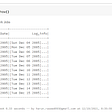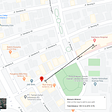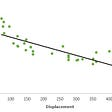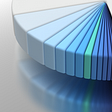# Spotify Data Visualization and Analysis using Python

## Data Analysis projects for the beginner as well as intermediate.

Most song lovers listen to songs on Spotify. It is one of the most popular song streaming platforms. And if you are a programmer then you know the relationship between code and songs. So, let’s start some analysis on Spotify with a cup of coffee.

# Code and Analysis

• Import the following libraries
`#for mathematical computationimport numpy as npimport pandas as pdimport scipy.stats as stats#for data visualizationimport seaborn as snsimport matplotlib.pyplot as pltfrom matplotlib.pyplot import figureimport plotly import plotly.express as px% matplotlib inline`
• Let’s load the data and take a sneak peek at the data. Download the dataset and add that to the path. After that render the first 5 data of the dataset.
`df = pd.read_csv("/content/spotify_dataset.csv", encoding='latin-1')df.head()`

Now run the cell, you will see something like this on-screen.

`#data infodf.info()#Check missing valuesdf.isnull().sum()`

Check out the null values in each column. We got lucky that there are no null values in our dataset.

• Number of times charted by artists
`#number of times charted by artistdf_numbercharted=df.groupby('Artist').sum().sort_values('Number of Times Charted', ascending=False)df_numbercharted=df_numbercharted.reset_index()df_numbercharted`

For this, we take an artist and sum the number of times charted and align each of them in descending order.

`px.bar(x='Artist', y='Number of Times Charted', data_frame=df_numbercharted.head(7), title="Top 7 Artists with Highes Number of Times Charted")`

When you run the cell, you will see something like the image above. Billie Elish tops the list of the highest number of times charted. The above bar chart has only the top 7 artists. You can check the top 10 or more artists. Just try to play with code.

• Correlations between the columns

Let’s see the correlations between the columns, and check if we can find anything interesting. For this, let’s first clean the data we have. After that, convert all the columns to numeric.

`#clean data firstdf=df.fillna('')df=df.replace(' ', '')df['Streams']=df['Streams'].str.replace(',','')#convet all numeric columns to numericdf[['Highest Charting Position', 'Number of Times Charted', 'Streams', 'Popularity', 'Danceability', 'Energy', 'Loudness', 'Speechiness',       'Acousticness', 'Liveness', 'Tempo', 'Duration (ms)', 'Valence',       ]] = df[['Highest Charting Position', 'Number of Times Charted', 'Streams','Popularity', 'Danceability', 'Energy', 'Loudness', 'Speechiness',       'Acousticness', 'Liveness', 'Tempo', 'Duration (ms)', 'Valence',       ]].apply(pd.to_numeric)`

Let’s also separate the year from the column “Release date” to be able to analyze its correlations.

`df['Release Year'] = pd.DatetimeIndex(df['Release Date']).year`

Now, plot the heatmap.

`%matplotlib inlinef,ax = plt.subplots(figsize=(14,10))sns.heatmap(df.corr(),annot = True,fmt = ".1f",ax = ax)plt.show()`

As we all know that Acoustic music is often quiet and requires careful listening. That’s why it makes a negative correlation with energy and loudness, which makes sense.

Now, in the code, “annot” is used to show the numbers in the cube. “fmt” is used for the numbers, if you set fmt=”0.2%” then in the cube numbers will appear in the form of percentage with 2 decimal places. Clearly, we don’t want that, because it makes the readability hazy.

• Danceability
`px.line(x='Release Year', y='Danceability', data_frame=df, title="Danceability over the course of the Year")`

Now, have a look at how danceability is changing over the years. When you run the cell with the above command, you will see something like this on-screen.

• Number of Times Charted correlates with years
`dfyear = df.groupby('Release Year').sum().sort_values('Number of Times Charted', ascending=False)dfyear=dfyear.reset_index()`

It’s the simple one, group the data by “Release Year” and sort them with the sum of “Number of Times charted” in each year.

Plot the graph.

`px.bar(x='Release Year', y='Number of Times Charted', data_frame=dfyear.head(7))`

Since 2021 is going on, we have fewer data for 2021. Most of the data come from the year 2020.

• 20 Most Popular Artists
`artistbypop = df.groupby('Artist').sum().sort_values('Popularity' ,ascending=False)[:20]artistbypop=artistbypop.reset_index()#plot the graphpx.bar(x='Artist', y='Popularity', data_frame=artistbypop)`

Here also, we did the same, we sort the Artists based on popularity. Taylor Swift tops the list followed by Juice WRLD and others. My favorite artist is in the ninth position.

• Most popular genres
`df['Genre']=df['Genre'].astype(str)df["Genre"][df["Genre"] == "[]"] = np.nandf["Genre"] = df["Genre"].fillna(0)#here we get rid of useless symbols to be able to separate genresdf.Genre=df.Genre.str.replace("[", "")df.Genre=df.Genre.str.replace("]", "")df.Genre=df.Genre.str.replace("'", "")#now we devide genre strings by commadf["Genre"] = df["Genre"].str.split(",")df=df.explode('Genre')df`

First, we get rid of useless symbols to be able to separate genres.

After that, divide genre strings by comma.

The next command separates rows based on genres. Each song that has more than one genre will have multiple rows with one genre in each row. For example, if a song has 2 genres then the same song will have 2 rows with different genres in each row.

Now simply plot the pie chart of the 30 most popular genres.

`fig = plt.figure(figsize = (10, 10))ax = fig.subplots()df.Genre.value_counts()[:30].plot(ax=ax, kind = "pie")ax.set_ylabel("")ax.set_title("Top 30 most popular genres")plt.show()`

Well, That’s it. Congrats, you analyzed the Spotify dataset. You can dig more on your own. Because you can do a lot with data. And the information you get is valuable.

Full Github code and dataset access are here.

--

--

--

## More from Geek Culture

A new tech publication by Start it up (https://medium.com/swlh).

## show(),collect(),take() in Databricks## #5 Visual programming with Orange tool## Want benefit from Big Data? Then ask Right Questions## Reverse address to latitude and longitude using here API## Training Time Series Forecasting Models in PyTorch## Twitter Predicting a Crashing Victory for One of the US Candidates## Regression Modelsninza7.me

## Prediction: Excel Users will need to learn data science to remain relevant## Unicorn Startups Data Analysis and Visualization in Python## Web Scraping Wikipedia Table using BeautifulSoup and pandas.read_html()## Exploratory Data Analysis in Python